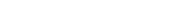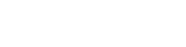GED Math: Factoring Polynomials

Factoring is a method for “un-multiplying” a polynomial. In other words, factoring is the method of breaking down a polynomial into smaller parts (called factors) whose product is equal to the original polynomial.

Factoring is just one topic in algebra. For more, check out: GED Algebra Prep.

Greatest Common Factors

When you need to factor a polynomial, first look for a greatest common factor (GCF). The GCF of a polynomial is an expression that divides into every term (with no remainder), and such that no greater such term exists. It’s made up of two parts:

1. The GCF of the numerical coefficients. This could be 1 if there are no larger factors common to all coefficients.
2. Variable factor with the least power among all variable powers showing up in the polynomial. This would be 1 if there is a constant term.

For example, the polynomial 12x7 + 18x3 has GCF = 6x3. On the other hand, the GCF of the polynomial 3x2 – 7x + 10 is simply 1, because there are no nontrivial numeric or variable factors common to all three terms.

After finding the GCF, if it’s greater 1, then you should factor out by it (When GCF=1, factoring out by it doesn’t change the polynomial). Divide each term by the GCF to determine what remains.

So you would factor the first example as follows:   12x7 + 18x3 = 6x3( 2x4 + 3).

Difference of Squares

There is a famous and very useful formula in Algebra called Difference of Squares.It’s easy to apply, but only works in specific situations: two-term polynomials in which both terms are perfect squares.

For example, 4x2 – 81 can be factored by Difference of Squares. The result is: (2x – 9)(2x + 9). (This is because (2x)2 = 4x2 and 92 = 81.)

However, 3x2 – 12 doesn’t quite fit the rule… yet. On the other hand, there is a GCF. Let’s first factor out by the GCF of 3.

3x2 – 12 = 3(x2 – 4)

Now we can use Difference of Squares on the remaining polynomial.

… = 3(x – 2)(x + 2)

Don’t Make up Your Own Rules

It’s very important to use the rules correctly. For example, there is no “Sum of Squares” factoring rule.

So don’t try to factor something like x2 + 16 into factors such as (x – 4) or (x + 4). It just doesn’t work that way. (In fact, sums of squares do not generally factor down any further.)

Perfect Square Trinomials

There are two related rules involving polynomials that factor into a pair of the same factors — in other words, polynomials that are themselves perfect squares. This rule applies only to trinomials, polynomials with exactly three terms.In practice, these might be tricky to spot. But first look at the constant term as a clue. If the constant term is a perfect square, then you just have to check the middle term to see if it fits the pattern.

For example, consider x2 – 22x + 121. We can see that 121 = 112. So there’s a chance that we’re dealing with a perfect square trinomial with a = x and b = 11. Check the middle term against 2ab.

2ab = 2(x)(11) = 22x

That’s exactly what shows up in our middle term, so we can use the rule! Check the sign on the middle term — it’s negative. Therefore,

x2 – 22x + 121 = (x – 11)2

Trinomial Factoring

Most trinomials are not perfect squares, so we need to have a general method for factoring them. There are two cases:

• Leading coefficient = 1. That is, the polynomial looks like: x2 + bx + c.
• Leading coefficient > 1. In other words, the polynomial has the form: ax2 + bx + c, where a > 1.

This is the case in which the polynomial has the form x2 + bx + c. Because the first term is just x2, we know that the factors must look like this:

(x + __)(x + __)

Your job is to fill in the blanks. We need to find two numbers that multiply to give you c and add up to b.

Let’s try it with a simple example. Factor x2 + 7x + 12.

Here, b = 7 and c = 12. What are the factors of 12? Write them in pairs and then put their sums in the third column of a table. (By the way, if you need a refresher on finding factors of a number, check out: GED Math: Factors, Multiples, and Primes.)

Factor 1Factor 2Sum
11213
268
347

We can see that 3 and 4 do the trick because 3 × 4 = 12 and 3 + 4 = 7. Therefore,

x2 + 7x + 12 = (x + 3)(x + 4).

The method also works when b and/or c are negative.

• If c > 0, then the two factors must have the same sign. They are both positive if b > 0, and both negative if b < 0.
• If c < 0, then the two factors must have opposite signs. In this case, if b > 0, then the larger factor (in absolute value) has the positive sign, while if b < 0, then the larger factor has the negative sign.

For example, let’s factor x2x – 20.

We need the factors of 20. Because c = -20 is negative, and b = -1 is also negative, the signs of the two factors will be opposite, with the minus on the larger factor.

Factor 1Factor 2Sum
1-20-19
2-10-8
4-5-1

It looks like 4 and -5 do the job. Therefore,

x2x – 20 = (x + 4)(x – 5).

Now if the leading coefficient is larger than 1, the process is more involved. We have to keep track of much more information, and there are more combinations to consider.

One method that seems to keep everything organized is the so-called box method. Here’s how it works.

To factor trinomials of the form ax2 + bx + c, in which there is no GCF:

1. First multiply a × c.
2. Next, find two numbers that will multiply to ac and add to b. (This step is just as in the coefficient=1 case, except that we use ac in place of c.)
3. Build a 2 by 2 box. Place the term ax2 in the upper left and c in the lower right. Then fill the other two boxes with the two terms that would add to get the middle term bx (using your numbers from step 2). It doesnt’t matter which order you put the last two terms in.
4. Factor out the GCF of each row, placing the result outside the box on the left.
5. Factor out the GCF of each column, placing the result outside the box below.
6. The terms to the left of the box form one factor, and the terms below the box form the second factor. Just be sure to put plus signs between the terms.

Let’s see how it works in practice.

Factor 4x2 + 5x – 6.

Here, we have ac = 4 × (-6) = -24. Since the result is negative, we need our factors to have opposition sign. But since the middle term is positive, the minus goes onto the smaller factor.

Factor 1Factor 2Sum
-12423
-21210
-385
-462

We can see that -3 and 8 will add to 5. That means that 5x = -3x + 8x.

Now let’s build the box.Here, the black terms were filled in first. 4x2 is the leading term of the polynomial (upper left), -6 is the constant term (lower right). Then with our splitting of 5x into -3x and 8x, you should place those terms into the other boxes as shown.

The GCF of the first row is x, and the GCF of the second row is 2. Those terms are in red on the left side. Working down the columns, the corresponding GCFs are 4x and -3, shown below the main table.

Therefore, reading the factors off of the table, we have:

4x2 + 5x – 6 = (x + 2)(4x – 3).

Factoring by Grouping

So far, we’ve seen factoring of binomials and trinomials. What about polynomials with four or more terms? There are not a lot of options available in those cases. Certainly look for a GCF. In fact, that should be your initial step in every factoring problem. But as a last resort, you might try factoring by grouping.

To factor by grouping:

1. First factor out a GCF if there is one.
2. Group the terms in pairs.
3. Find a GCF and factor each pair.
4. If the expressions in parentheses match, then that expression can be factored out.
5. Determine if there is any further factoring that can be done in the resulting expression.

For example, we can use factoring by grouping on the following polynomial:

4x3 + 12x2x – 3

First, look for a GCF. The GCF is 1, meaning that there is nothing to factor out from all of the terms at once.

Next, group into pairs and factor each pair. Be careful with negatives!

(4x3 + 12x2) + ( –x – 3) = 4x2(x + 3) + (-1)(x + 3)

We’re in luck! Both pairs have the expression (x + 3) in common. That means we can continue the factoring process. Pull out this common factor from both terms.

… = (4x2 – 1)(x + 3)

Finally, look for further factoring. The first group can be broken down by Difference of Squares.

… = (2x – 1)(2x + 1)(x + 3)

Practice Problems

Now’s your chance to check your progress! Factor each of the following polynomials. Answers will be given below.

1. x2 – 8x – 9
2. t2 – 25
3. t2 + 25
4. 2y2 + 8y4
5. 32x2 – 98
6. x2 + 12x + 36
7. z3 + 11z2 – 4z – 44
8. 2x2 + 2x – 4
9. x3 – 4x2 – 21x
10. 6x2 – 7x – 31. (x + 1)(x – 9)
2. (t – 5)(t + 5)
3. t2 + 25 (does not factor further)
4. 2y2(1 + 4y2)
5. 2(4x – 7)(4x + 7)
6. (x + 6)2
7. (z – 2)(z + 2)(z + 11)
8. 2(x – 1)(x + 2)
9. x(x + 3)(x – 7)
10. (3x + 1)(2x – 3)

Author

•Shaun earned his Ph. D. in mathematics from The Ohio State University in 2008 (Go Bucks!!). He received his BA in Mathematics with a minor in computer science from Oberlin College in 2002. In addition, Shaun earned a B. Mus. from the Oberlin Conservatory in the same year, with a major in music composition. Shaun still loves music -- almost as much as math! -- and he (thinks he) can play piano, guitar, and bass. Shaun has taught and tutored students in mathematics for about a decade, and hopes his experience can help you to succeed!